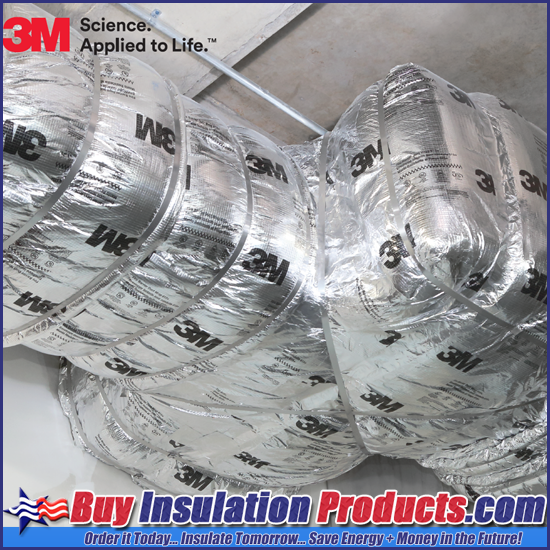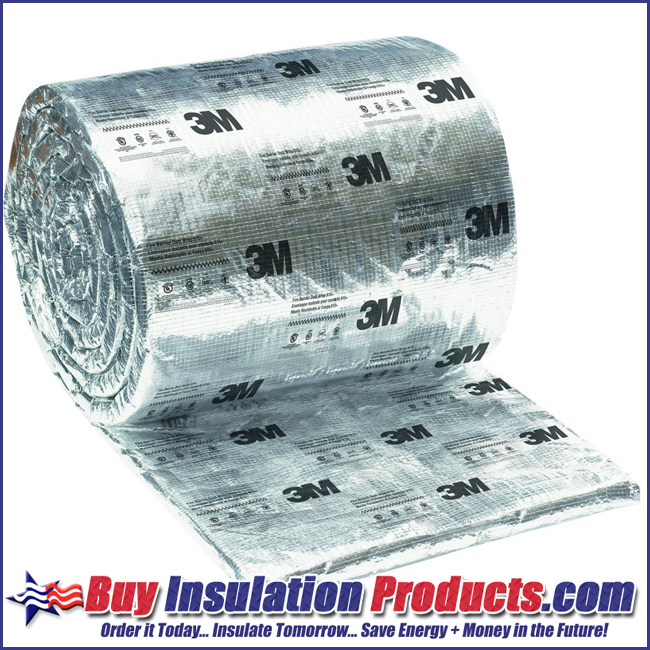## Estimating How Much 3M Fire Barrier Duct 615+ Duct Wrap is Needed

Posted by BIP on 12th May 2021

3M™ Fire Barrier Duct Wrap 615+ is an ideal fire resistive enclosure for commercial kitchen grease ducts and ventilation air ducts. It is a proven alternative to a 1- or 2-hour fire-resistant rated shaft enclosures for grease ducts and provides zero clearance to combustible construction throughout the entire enclosure system (per ICC-ES ESR-1255).  This blog post will provide the information on how to estimate how much 3M Fire Barrier Duct Wrap is needed for a Grease Duct with double layer installation.MATERIAL ESTIMATION FOR SQUARE OR RECTANGULAR DUCT

• LAYER 1 = (2(W+H)+13)/12 = SF/LF of Duct
• H= Height of Duct
• W= Width of Duct
• Example: 20 linear feet of a rectangular duct which is 12" x 18"
• (2(12+18)+13/12 = 6.083 square feet per linear foot
• 6.083 x 20 linear feet = 121.66 square feet of material are needed for the first layer.
• LAYER 2 = (2(W+H)+19)/10.667 = SF/LF of Duct
• Example: 20 linear feet of a rectangular duct which is 12" x 18"
• (2(12+18)+19/10.667 = 7.41 square feet per linear feet
• 7.41 x 20 linear feet = 148.2 square feet of material are needed for the second layer.
• 1ST LAYER = 121.66 sf*
• 2ND LAYER = 148.2 sf*
• TOTAL MATERIAL = 270 sf*
• *These calculations do not take any scrap material into consideration.MATERIAL ESTIMATION FOR CIRCULAR DUCT

• LAYER 1 = (π (D+3)+3)/12 = SF/LF of Duct
• π = Pi which is 3.14
• D = Diameter of the Duct
• Example: 20 linear feet of a circular duct which is 18" in diameter
• (3.14 (18+3)+3)/12 = 5.75 square feet per linear foot
• 5.75 x 20 linear feet = 115 square feet of material are needed for the first layer.
• LAYER 2 = (π (D+7.5)+3)/10.667 = SF/LF of Duct
• Example: 20 linear feet of a circular duct which is 18" in diameter
• (3.14 (18+7.5)+3)/10.667 = 7.79 square feet per linear foot
• 7.79 x 20 linear feet = 155.8 square feet of material are needed for the second layer.
• 1ST LAYER = 115 sf*
• 2ND LAYER = 155.8 sf*
• TOTAL MATERIAL = 271 sf*
• *These calculations do not take any scrap material into consideration.

If you have any questions or need additional explanation of the estimation process for 3M Fire Barrier Duct Wrap do not hesitate to ask our customer service department for help and quotations.

Now that you know how to properly estimate how much 3M Fire Barrier Duct Wrap you need for your project, you will want to read our blog post on how to properly install the duct wrap to the grease duct to pass your fire inspection.  Proper installation is imperative to providing a fire rating for the duct work which may prevent a future grease duct fire.